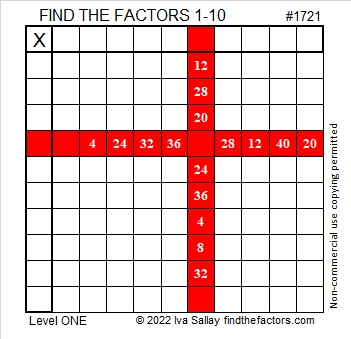# 1721 A Gift With Multiple Treasures

Contents

### Today’s Puzzle:

‘Tis the season of giving, and here’s a gift with multiple treasures inside. Write the numbers from 1 to 10 in both the first column and the top row so that the given clues are the products of the numbers you write. After you find the factors, you can complete the puzzle by finding all of the products.### Factors of 1721:

• 1721 is a prime number.
• Prime factorization: 1721 is prime.
• 1721 has no exponents greater than 1 in its prime factorization, so √1721 cannot be simplified.
• The exponent in the prime factorization is 1. Adding one to that exponent we get (1 + 1) = 2. Therefore 1721 has exactly 2 factors.
• The factors of 1721 are outlined with their factor pair partners in the graphic below.How do we know that 1721 is a prime number? If 1721 were not a prime number, then it would be divisible by at least one prime number less than or equal to √1721. Since 1721 cannot be divided evenly by 2, 3, 5, 7, 11, 13, 17, 19, 23, 29, 31, 37, or 41, we know that 1721 is a prime number.

### More About the Number 1721:

1721 is the sum of two squares:
40² + 11² = 1721.

Here’s another way we know that 1721 is a prime number: Since its last two digits divided by 4 leave a remainder of 1, and 40² + 11² = 1721 with 40 and 11 having no common prime factors, 1721 will be prime unless it is divisible by a prime number Pythagorean triple hypotenuse less than or equal to √1721. Since 1721 is not divisible by 5, 13, 17, 29, 37, or 41, we know that 1721 is a prime number.

1721 and 1723 are twin primes.

This site uses Akismet to reduce spam. Learn how your comment data is processed.